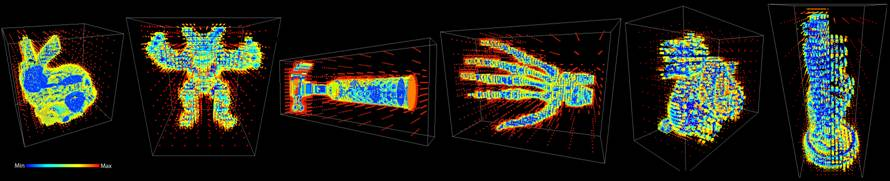Exact and Adaptive Signed Distance Fields

Computation for Rigid and Deformable Models on GPUs

 Fuchang Liu and Young J. Kim Department of Computer Science & Engineering Ewha Womans University, Seoul, Korea

 Accepted by IEEE Transactions on Visualization and Computer Graphics,2014 [paper][Errata]

# Abstract

Most techniques for real-time construction of a signed distance field, whether on a CPU or GPU, involve approximate distances. We use a GPU to build an exact adaptive distance field, constructed from an octree by using the Morton code. We use rectangle-swept spheres to construct a bounding volume hierarchy (BVH) around a triangulated model. To speed up BVH construction, we can use a multi-BVH structure to improve the workload balance between GPU processors. An upper bound on distance to the model provided by the octree itself allows us to reduce the number of BVHs involved in determining the distances from the centers of octree nodes at successively lower levels, prior to an exact distance query involving the remaining BVHs. Distance fields can be constructed 35-64 times as fast as a serial CPU implementation of a similar algorithm, allowing us to simulate a piece of fabric interacting with the Stanford Bunny at 20 frames per second.

# Benchmarking Scenarios

1. Rigid Models:

We applied our GPU-based parallel
algorithm to six geometric models, and generated adaptive distance fields with resolutions from 8^3 to 1024^3. We compared our GPU approach to a CPU implementation using the PQP proximity library. Our GPU implementation is 35 to 64 faster.Distance field computation times on a GPU and a CPU.   We also compared the performance of a single BVH with a multi-BVH.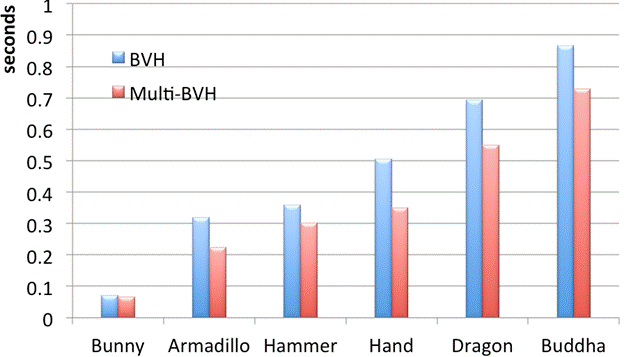Distance field computation times using a single-BVH and a multi-BVH. The times include adaptive sampling, BVH construction and distance queries.

2. Deformable Models:

We linked our distance field algorithm to a physics simulation. We inflated the Bunny (69K triangles) and dropped a cloth (4K vertices) around it. In this benchmark, the distance fields are recomputed for every simulation step. We computed responsive forces using penetration depth obtained from the distance field of bunny. We simulated the response of the cloth against the bunny at 20 frames per second.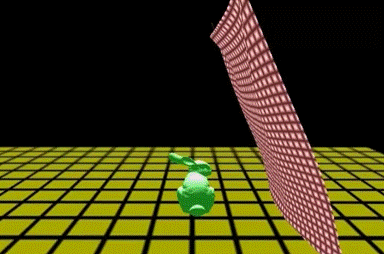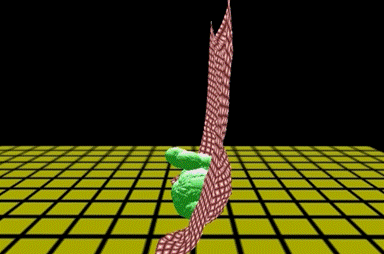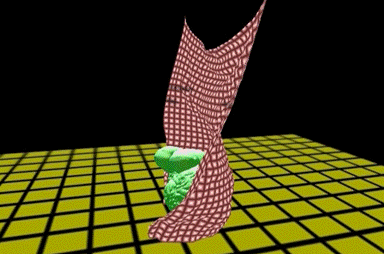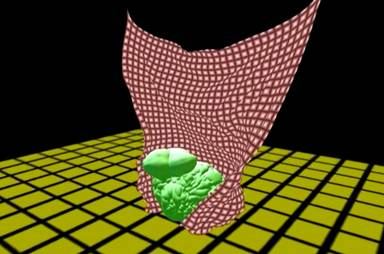Simulation of a cloth falling around the Stanford Bunny.